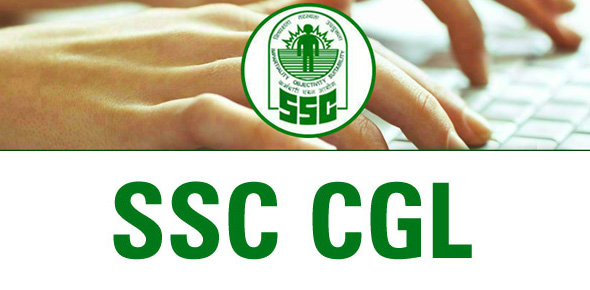# SSC CGL Exam(Tier-I):Reasoning:

20 Questions | Total Attempts: 17SettingsThe SSC CGL reasoning exam below tests your thinking capacity and ability. It basically tests on your ability to note and follow past patterns to come up with a correct answer of unrelated things. All the best.

• 1.
If GOLD is written as IQNF, how WIND can be written in the code?
• A.

YKPF

• B.

VHMC

• C.

XJOE

• D.

DNIW

• 2.
If A = 1, PAT = 37, then TAP =?
• A.

73

• B.

37

• C.

36

• D.

38

• 3.
If D = 4, BAD = 7, then what is the value of ANT =?
• A.

8

• B.

17

• C.

35

• D.

37

• 4.
If HKUJ means FISH, what does UVCD mean?
• A.

STAR

• B.

STAB

• C.

STAL

• D.

STAK

• 5.
If CONSCIOUSLY is written as PEBNPJEXNKM, then SOIL is written as:
• A.

NEKJ

• B.

NEJK

• C.

JENK

• D.

ENJK

• 6.
If C = 3 and FEAR is coded as 30, then what will be the code number for HAIR?
• A.

35

• B.

36

• C.

30

• D.

33

• 7.
If Z = 26, NET = 39, then NUT =?
• A.

50

• B.

53

• C.

55

• D.

56

• 8.
If F = 6, MAT = 34, then how much is CAR?
• A.

21

• B.

22

• C.

25

• D.

28

• 9.
If NOIDA is written as STNIF, how MEERUT can be written in that code?
• A.

QIIVYX

• B.

RJJWZV

• C.

RJJWZY

• D.

RIIVYX

• 10.
In a certain code FORGET is written as DPPHCU, how would DOCTOR be written in that code?
• A.

BPAUMS

• B.

BPAUPS

• C.

EMDRPP

• D.

BPARPP

• 11.
In a certain code DECEMBER is written as ERMBCEDE, in that code which word will be written as ERMBVENO?
• A.

SEPTEMBER

• B.

AUGUST

• C.

NOVEMBER

• D.

OCTOBER

• 12.
If RED is coded as 6720, then how GREEN would be coded?
• A.

9207716

• B.

1677199

• C.

1677209

• D.

16717209

• 13.
If ROSE is written as TQUG, how BISCUIT can be written in that code?
• A.

CJTDVJU

• B.

DKVEWKV

• C.

DKUEWKY

• D.

DKUEWKV

• 14.
If the word MENTAL is written as LNDFM-OSUZBKM, then how would the word TEST be written in that code?
• A.

UVFGTUUV

• B.

RSCDQRRS

• C.

SUDFQRSU

• D.

SUDFRTSU

• 15.
If TYPEWRITER is written as GBKVDIRGVI, how STENO can be written in that code?
• A.

LMVGH

• B.

HGVML

• C.

LMHGV

• D.

HVLGM

• 16.
If KASHMIR is written as 8142753, how RIMSHAK can be written in that code?
• A.

3574218

• B.

3571842

• C.

3521478

• D.

3574812

• 17.
If 'A' = 26, SUN = 27 then CAT=?
• A.

24

• B.

57

• C.

58

• D.

27

• 18.
If in a code language "ORGANISATION" is written as "CBDWLQJWYQCL" and "OPERA-TION" is written as "CXFBWYQCL", how is "SEPARATION" coded?
• A.

EJXEBEYQCL

• B.

JFQYWBCXQL

• C.

JFXWBWYQCL

• D.

QCLYWBFXJE

• 19.
If A = 2, M = 26 and Z = 52 then BET =?
• A.

44

• B.

54

• C.

64

• D.

72

• 20.
In a particular way of coding, the word CENTRAL is coded as ABCDEFG and PLAN-ETARIUM as HGFCB-DFEIJK. With the same coding how can we express the word LANTERN?
• A.

GFCDFEG

• B.

GFCDBEC

• C.

GFCDEFG

• D.

GFCDBEB